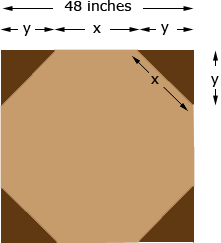I have a 48 inch square piece of wood that I need to cut into an octagon, help. What's an easy way to just measure and cut it. Thank you Freddie Freddie, I drew a diagram and labeled some lengths.From the diagram x + 2 y = 48 The triangle in the upper right that is to be cut off is a right triangle and hence from Pythagoras theorem y2 + y2 = x2 Thus 2 y2 = x2 and √2 y = x. Substituting into the first equation gives √2 y + 2 y = 48 so y = 48/(√2 + 2)  = 14.06 inches or 14 1/16 inches. Penny